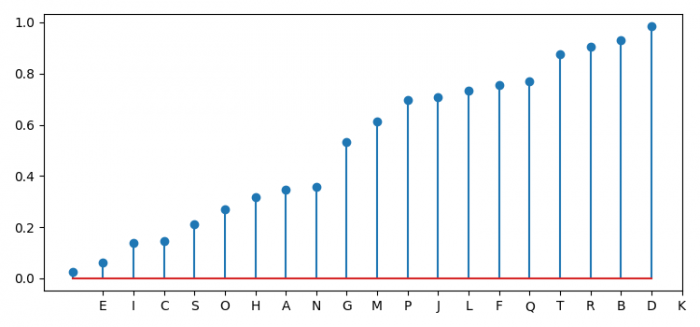# How to make a simple lollipop plot in Matplotlib?

To make a simple lollipop plot in Matplotlib, we can take the following steps −

• Set the figure size and adjust the padding between and around the subplots.

• Make a dataframe, df, of two-dimensional, size-mutable, potentially heterogeneous tabular data.

• Make an ordered dataframe, using sort_values().

• Make a list in the range of dataframe index.

• Create a stem plot, using the ordered dataframe.

• Set xticks and labels using xticks() method.

• To display the figure, use show() method.

## Example

import numpy as np
from matplotlib import pyplot as plt
import pandas as pd

plt.rcParams["figure.figsize"] = [7.50, 3.50]
plt.rcParams["figure.autolayout"] = True

df = pd.DataFrame({'group': list(map(chr, range(65, 85))), 'values': np.random.uniform(size=20)})

ordered_df = df.sort_values(by='values')

my_range = range(1, len(df.index) + 1)

plt.stem(ordered_df['values'])

plt.xticks(my_range, ordered_df['group'])

plt.show()

## OutputUpdated on: 23-Sep-2021

278 Views